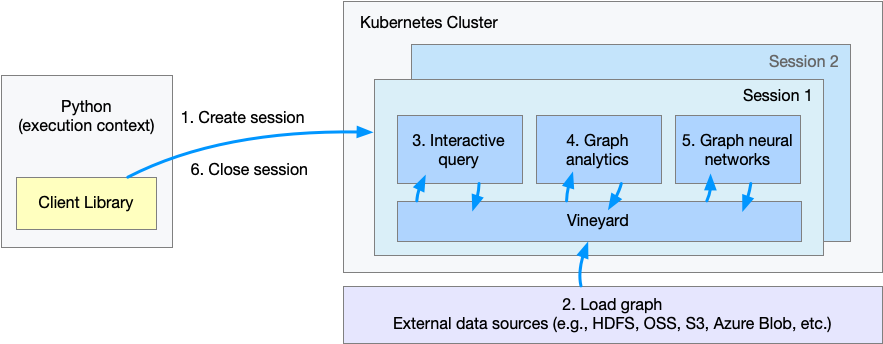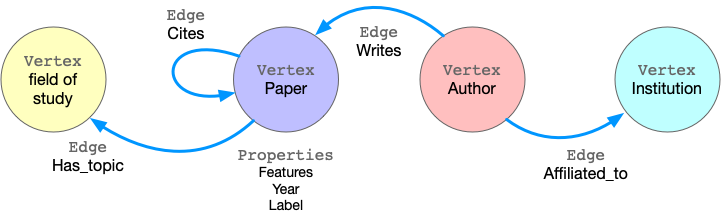# 快速上手#

GraphScope 做为一站式图计算系统，可以处理图的交互式查询、图分析和图学习任务, 并且具有易于使用、高性能和良好的可扩展性等特点。

## 示例: 论文引用网络中的节点分类任务#

ogbn-mag 数据集 是由微软学术关系图（Microsoft Academic Graph）的子集组成的异构图网络。 该图中包含4种类型的实体（即论文、作者、机构和研究领域），以及连接两个实体的四种类型的有向关系边。• 步骤 1. 建立一个会话（session），为 GraphScope 创建一个工作空间；

• 步骤 2. 定义和载入图；

• 步骤 3. 通过 gremlin 交互式查询图；

• 步骤 4. 执行图算法做图分析；

• 步骤 5. 执行基于图数据的机器学习任务；

• 步骤 6. 关闭会话。

## 创建会话#

import os
import graphscope

# assume we mount ~/test_data to /testingdata in pods, and ogbn_mag_small within.
k8s_volumes = {
"data": {
"type": "hostPath",
"field": {
"path": os.path.expanduser("~/test_data/"),
"type": "Directory"
},
"mounts": {
"mountPath": "/testingdata"
}
}
}

sess = graphscope.session(k8s_volumes=k8s_volumes)


sess = graphscope.session(k8s_volumes=k8s_volumes, k8s_service_type="LoadBalancer")


GraphScope 以属性图（property graph）建模图数据。属性图中，点和边都有一个标签（label），不同的标签有不同的属性（property）。 以 ogbn-mag 为例，下图展示了属性图的模型。g = sess.g()
g = (
"author_affiliated_with_institution.csv",
label="affiliated",
src_label="author",
dst_label="institution",
)
"paper_has_topic_field_of_study.csv",
label="hasTopic",
src_label="paper",
dst_label="field_of_study",
)
"paper_cites_paper.csv",
label="cites",
src_label="paper",
dst_label="paper",
)
"author_writes_paper.csv",
label="writes",
src_label="author",
dst_label="paper",
)
)


from graphscope.dataset import load_ogbn_mag



## 交互式查询#

# get the entrypoint for submitting Gremlin queries on graph g.
interactive = sess.gremlin(g)

# check the total node_num and edge_num
node_num = interactive.execute("g.V().count()").one()
edge_num = interactive.execute("g.E().count()").one()

# count the number of papers two authors (with id 2 and 4307) have co-authored.
papers = interactive.execute("g.V().has('author', 'id', 2).out('writes') \
.where(__.in('writes').has('id', 4307)).count()").one()


## 图分析#

# exact a subgraph of publication within a time range
sub_graph = interactive.subgraph("g.V().has('year', gte(2014).and(lte(2020))).outE('cites')")

# project the projected graph to simple graph.
simple_g = sub_graph.project(vertices={"paper": []}, edges={"cites": []})

ret1 = graphscope.k_core(simple_g, k=5)
ret2 = graphscope.triangles(simple_g)

# add the results as new columns to the citation graph


## 图神经网络 (GNNs)#

# define the features for learning
paper_features = [f"feat_{i}" for i in range(128)]

paper_features.append("kcore")
paper_features.append("tc")

# launch a learning engine.
lg = sess.graphlearn(sub_graph, nodes=[("paper", paper_features)],
edges=[("paper", "cites", "paper")],
gen_labels=[
("train", "paper", 100, (0, 75)),
("val", "paper", 100, (75, 85)),
("test", "paper", 100, (85, 100))
])


# Note: Here we use tensorflow as NN backend to train GNN model. so please
# install tensorflow.
try:
# https://www.tensorflow.org/guide/migrate
import tensorflow.compat.v1 as tf
tf.disable_v2_behavior()
except ImportError:
import tensorflow as tf

import graphscope.learning
from graphscope.learning.examples import EgoGraphSAGE
from graphscope.learning.examples.tf.trainer import LocalTrainer

# supervised GCN.
def train_gcn(graph, node_type, edge_type, class_num, features_num,
hops_num=2, nbrs_num=[25, 10], epochs=2,
hidden_dim=256, in_drop_rate=0.5, learning_rate=0.01,
):
graphscope.learning.reset_default_tf_graph()

dimensions = [features_num] + [hidden_dim] * (hops_num - 1) + [class_num]
model = EgoGraphSAGE(dimensions, act_func=tf.nn.relu, dropout=in_drop_rate)

# prepare train dataset
node_type=node_type, edge_type=edge_type, nbrs_num=nbrs_num, hops_num=hops_num,
)
train_embedding = model.forward(train_data.src_ego)
train_labels = train_data.src_ego.src.labels
loss = tf.reduce_mean(
tf.nn.sparse_softmax_cross_entropy_with_logits(
labels=train_labels, logits=train_embedding,
)
)

# prepare test dataset
node_type=node_type, edge_type=edge_type, nbrs_num=nbrs_num, hops_num=hops_num,
)
test_embedding = model.forward(test_data.src_ego)
test_labels = test_data.src_ego.src.labels
test_indices = tf.math.argmax(test_embedding, 1, output_type=tf.int32)
test_acc = tf.div(
tf.reduce_sum(tf.cast(tf.math.equal(test_indices, test_labels), tf.float32)),
tf.cast(tf.shape(test_labels), tf.float32),
)

# train and test
trainer = LocalTrainer()
trainer.train(train_data.iterator, loss, optimizer, epochs=epochs)
trainer.test(test_data.iterator, test_acc)

train_gcn(lg, node_type="paper", edge_type="cites",
class_num=349,  # output dimension
features_num=130,  # input dimension, 128 + kcore + triangle count
)


## 关闭会话#

sess.close()